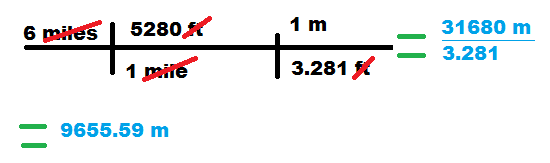Unit conversion question

Homework Statement

The person fell from the plane 6 miles and survived the fall.
What is the distance the person fell in metres?

1m=3.281ft
1mi=5280ft
1ft=0.3048m

The Attempt at a Solution

I figured if 1 mi = 5280ft
then 6 miles must be : 6(5280ft)=31, 680ft.
I also figured if 1ft = 0.3048m then 31, 680 * 0.3048 should get the distance in metres to be
9,656.06metres. The book says 10, 159m is the answer, so can anyone help me here please? Is it possible the book is wrong?!

Last edited:

tiny-tim
Homework Helper
Hi 1irishman!Your answer looks right to me.Borek
Mentor
Funny.

10159/6 = 1693.17 meters per mile

1 statute mile = 1609.344 meters

Looks to me like someone ate 0 when calculating the answer.